# Plasma Species

The plasma is assumed to consist of three (charged) species; namely, electrons (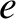), majority ions (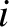), and impurity ions (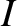). The charges of the three species are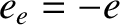,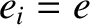, and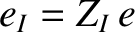, respectively, whereis the magnitude of the electron charge. Quasi-neutrality  demands that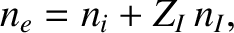(A.1)

where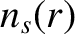is the species-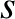number density. Here,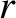is the flux-surface label introduced in Section 14.2. Let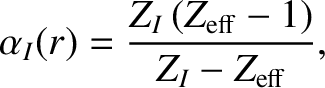(A.2)

where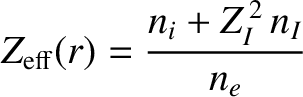(A.3)

is the effective ion charge number. (See Section 1.6.) It follows that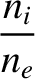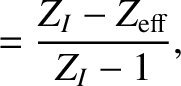(A.4)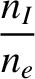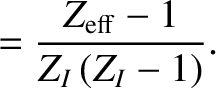(A.5)

Finally, let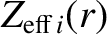(A.6)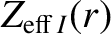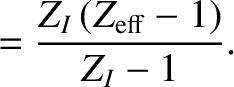(A.7)

Note that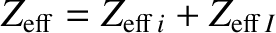.